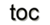Next: 2.2 Finite Volumes Up: 2. Numerical Discretization Schemes Previous: 2. Numerical Discretization Schemes

Subsections

# 2.1 Generic Discretization Concepts

This section gives an overview of the basic concepts for discretization schemes in scientific computing. The most important mechanisms are introduced and are then later converted into applicable and well known discretization schemes, such as the above mentioned finite volumes, finite elements, and finite differences. The purpose of this section is to unify different discretization schemes to a common kernel which is then directly converted to software design concepts. The concepts for discretization are mostly derived from an abstract reference discretization scheme , which is not a full discretization scheme by itself. Here only the necessary concepts for time-dependent electromagnetic problems which are discussed in subsequent chapters, are introduced, with some additional concepts from literature [32,24,33,36]

## 2.1.1 Domain Discretization

The simulation domain, either a simple space or a more complex space-time, is discretized by means of two dual oriented cell complexes for the primary and secondary mesh. The two cell complexes and therefore the primary and secondary mesh are not required to be physically or geometrically distinct. The logical separation is required to distinguish the different cochains/differential forms only. With dual cell complexes, the attribution of boundary conditions and the treatment of material discontinuities can be greatly simplified . The use of non-dual cell complexes, e.g., operator adjointness, is more complex to obtain. The primary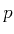-cells are identified by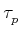whereas the corresponding secondary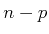-cells are identified by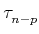and the default orientation, as given in Figure 2.1 for three dimensions.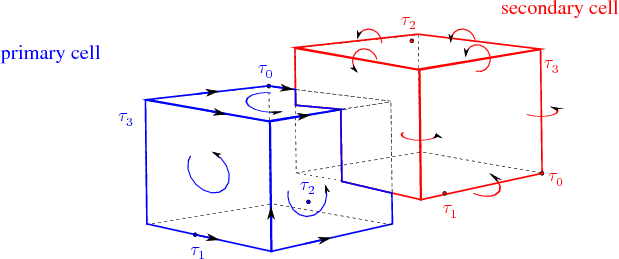Time is also subdivided into two dual cell complexes. Here the 0-cells are time instants indexed with increasing time. The time interval between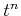to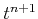is indexed as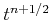, similar to the formulation used by Yee (see Section 2.4.2). The secondary cells are given byand depicted in Figure 2.2.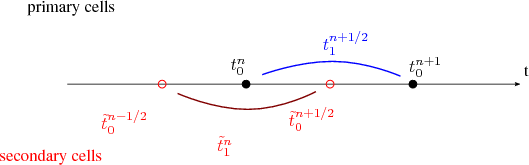The time-dependent equation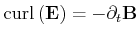(2.2)

can then be interpreted as a higher dimensional object of the spatial domain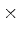the time domain: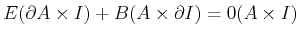(2.3)

A graphical representation is given in Figure 2.3 where two 0-cells are used for the corresponding time steps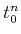and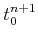and one 1-cell for the time interval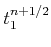. The corresponding quantity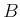is attached to the time interval, whereas the quantity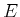corresponds to the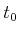cells.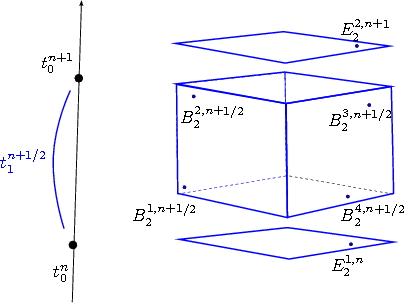This topological time stepping was proven  to be consistent with the conservation of charge as well as to preserve the absence of sources for the Faraday and Amperé laws. Based on this unique topological time stepping and on various discrete constitutive links, a variety of numerical time-stepping procedures, including explicit and implicit ones, can be derived.

## 2.1.2 Topological Equations

Balance or conservation equations can be written in local or global forms [24,33], e.g.:(2.4)

where the left equation represents a local or differential formulation and the right equation represents a global or discrete form. The discretization of the given relation means that the equation must be written for domains of finite extension. For topological equations this transition takes place without any error of approximation . The balance equation by itself does not require uniform fields, homogeneous materials or any other conditions. Various laws which can be described by topological equations are intrinsically discrete. They state a balance of global quantities whose validity does not depend on metrical or material properties and is therefore invariant for very general transformations. This gives them topological significance and the name topological equation was used  to express the idea of invariance under arbitrary homeomorphic transformations. For a topological equation, the discrete or global version appears as the fundamental one, with the differential statement resulting from it if additional requirements are fulfilled.

## 2.1.3 Constitutive Relation Discretization

Discretizing constitutive relations determines the link between various cochains which represents the field quantities approximated by the local constitutive equations. This step of discretization offers a great number of different choices .

### 2.1.3.1 Field Function Reconstruction and Projection

For most of the finite volume and finite element schemes, a field projection is used by: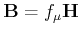(2.5)

In the discrete setting the field functions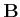and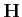do not belong to the problem's variable. Instead the magnetic flux cochain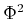and the magnetic field cochain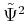are linked by the relation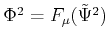.

From the cochaina field function is derived by a reconstruction operator: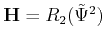(2.6)

Next, the local constitutive link, Equation 2.5, is used to derive. Then, the cochainhas to be obtained by means of a projection operator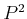, which produces a cochain for each field function: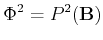(2.7)

The discrete constitutive link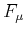is then finally given by: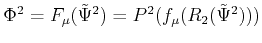(2.8)

A natural requirement for the reconstruction and projection operators is that for each cochain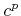the following relation holds true :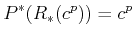(2.9)

To obtain a sparse matrix representation the reconstruction process is usually performed locally, so the value of the reconstructed field function in a particular point depends only on the values of the original cochain on the cells in a sufficiently small neighborhood of the point.

During the last decade a comprehensive framework for the reconstruction and projection operators, called mimetic discretization [63,64,65,66,37,25], was derived. This framework analyzes in detail how these two operators can be used to mimic the analytical and continuous nature of partial differential equation.Next: 2.2 Finite Volumes Up: 2. Numerical Discretization Schemes Previous: 2. Numerical Discretization Schemes

R. Heinzl: Concepts for Scientific Computing